### Help For Harbeck

Wednesday, February 18, 2009
Apparently Harbeck fell somewhere and bumped his head. Now he can't remember what he was going to teach us, but he remembers everything else. So he told us to help him, so here.. I go. Kind of. So, I'm suppose to explain what these "artifacts" are, so here I go!

All these pictures in the bottom are linked. How? The first, third and last picture are all connected to PYTHAGORAS, the second picture, because he was the one that created all of this. Pythagoras was the person that figured out the PYTHAGOREAN THEOREM. You can use the words "legs, hypotenuse, R.A.T, Greek, theorem" to help you with finding out and explaining your artifacts because each one connects to each picture. LEGS is connected with the Right Triangle. LEGS are the "A" and "B" on your Triangle, if you labelled it and "C" is the HYPOTENUSE (longest side of the Right Triangle). R.A.T is RIGHT ANGLE TRIANGLE, Greek is connected with Pythagoras because he is Greek, and also connects with the words THETA AND BETA. Theorem connects with every picture on this page, because it is the statement, theory of this all.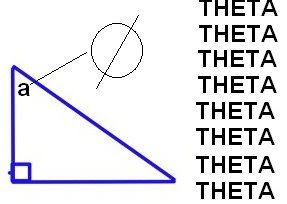The first shape is a RIGHT TRIANGLE. It is also known as R.A.T. (Right Angle Triangle). The right triangle has one 90 degree angle, which is on the corner. The first picture is the BETA, the second is the Theta. These angles both equal up to 90 degrees, which is called COMPLEMENTARY ANGLES. The longest line in the triangle is called the hypotenuse which is always labelled as C. The other two sides that make up the L, are called the legs which are always labelled as A or B.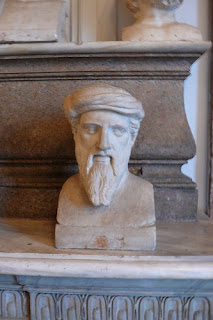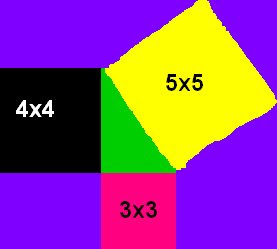The second picture is PYTHOGORAS a Greek Genius. Many people call him FATHER OF MATH. He made the PYTHAGOREAN THEOREM, somethng I'll explain later. He discovered the circumference of the world, and figured out that the Sun goes around the Earth. He is a Vegan, someone who doesn't eat things that are alive and a teacher. He loves Music just like how he loves Math. He believed that he could make a square out of four right triangle angles and that he could make a triangle out of three squares. This is his proof. > He used the PYTHAGOREAN THEOREM and made these squares. One 3x3 square, one 4x4 square and one 5x5 square which was made from both 3 and 4 squares. The outside boxes are the 3x3 boxes and the inside boxes are 4x4.The third picture is the PYTHAGOREAN THEOREM, I was talking about earlier. I think that this forumla is the relation to all three sides of the triangle. The first picture shows you how the pythagorean theorem works. I have a square of 3x3 and a square of 4x4. Harbeck gave us the assignment to put both squares together to make one square. The last square, 5x5 is the square that you should get when you put both squares together. The outside boxes are from the 3x3 square and the inside boxes are from the 4x4 square. The pythagorean theorem works for this, because 3x3 = 9, 4x4 = 16, and 5x5 = 25. 9+16=25. If you don't know the c2 side of a triangle (hypotenuse), then you find it by using what you know, and the pythagorean theorem. pretend one side of the triangle is 3 (side b) and one side of the triangle is 2 (a). the first thing you do is write out the pythagorean theorem. a2+b2=c2. then you write down what you know.You know that a is 2 squared and b is 3 squared. So you write it out. 3x3+2x2=c2. Simpify. 36+4=40. Then you find the square root of 40, which is 6.324. c2 is 6.324.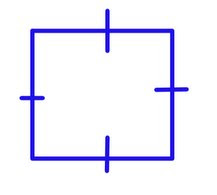The last picture is a square. It has 4 90 degree angles. All of the square equals up to 360 degrees. (90x4=360) You can make 2 right triangles out of this square by cutting it one of the corners. Like the first picture. The lines on the square tells you that all sides are equal, which means that this is a square and not a rectangle. If you know one side of the square, you know it all. You can find the area and perimeter. Pretend the square is 5 in length, it is also 5 in width. You can find the area by lxw, 5x5. The area is 25.

I care.. because I care. Education is important to everyone, I'm lucky to be attending a school without having to wait on the waiting list. I should appreciate it and do everything I can to successfully graduate it. (.. I don't know if I was actually suppose to answer this question or not.)

Math Video

I can't add this part to the PYTHAGOREAN THEOREM section because I have already published and made it into a wmv file. So, I'll just add the last part here.I couldn't find our HOW TO FIND C video, so I'll just write about it, just to get a few marks atleast. Let's pretend you have a triangle. Side A is 4 and side B is 5. We need to find C, the hypotenuse. So, let's write out the question. a^2+b^2=c^2. Now we fill in what we know. We know that A is 4, so 4^2 and B is 5. So, 5^2. 4^2+5^2=c^2. 4x4+5x5=c^2. Now we simplify it. 16+25=41. To find out what c is, we need to also find the square root of 41. 6.403 is the square root, so that's the measurement for 41.

Pythagoras Homework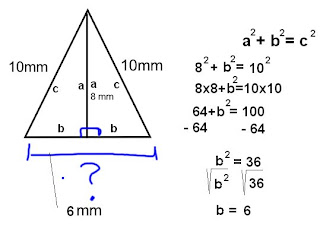This is the Math Homework we received from Harbeck on February 23. We are suppose to find the base, which is why there is a question mark on there. The second picture shows how you solve c, just click on it to make it larger. I know what a is (8mm), so I can use that in my equation. I know what c is, (10mm), I can also use that in my equation.The square is 225cm2. we find the square root, which is 15. Now we know that side b is 15, and side a is 15. since both are the same size. Now all we need to do is solve C using the Pythagorean Theorem. a2+b2=c2, 15x15+15x15=c2, 225+225=450. We need to find the square root of 450, which is 21.213. We now know that the Hypotenuse, which is always labelled as c is 21.213. we now try to find the perimeter. We multiply 21.213 by 4 or add 21.213 four times, because there are triangles in this picture, making 4 hypotenuse. 21.213x4=84.852. Then you multiply the legs which are labelled as a and b by 4 (or add 15 four times), because there are four triangles in this picture. 15x5=60. you then add them together. 60+84.852=144.852. the perimeter of this board is 144.852.